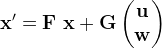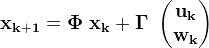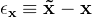PSAS/ MathSymbols

# Mathematical Symbols and Notation

## Notation used in State Space control and Kalman filtering

The basic state space formulation of a dynamic system expresses the system as a linear first order differential equation with respect to timeorThe first form is just a matrix representation of the system differential equations. The second form denotes a solution to those equations. As expressed here, the first form is written for continuous time systems while the second form is written for a discreet system. This is a common arrangement, but either form can be used for either system type.

Summary of State Space Symbols

Symbol Definition Name
x n×1 state vector
F n×n system matrix
Φ n×n state transition matrix
G n×q input matrix
Γ n×q discreet input matrix
u qu×1 input vector
w qw×1 process noise vector

The vector lengths of (u) and (w) are given as (qu) and (qw) respectively. The total length of the combined input vector is (q = qu + qw), which conforms correctly to the dimensions of the input matrix (G) or (Γ).

Perhaps awkwardly, in this formulation we have both distinguished and combined the input vector (u) and the process noise vector (w). The distinction is that (u) is usually treated as a controlled input, like a knob on a machine or the rudder of a ship. The process noise vector (w), though still an input to the system, is not under the control of any part of the system. The process noise input represents such things as random disturbances in the movement of a vehicle, or errors in modeling of the system.

An alternate approach is to write the two inputs as separate terms. This requires the introduction of a new matrix like so

• x' = F x + G u + L w

or

• xk+1 = Φ xk + Γ uk + Λ wk

The new matrices (L) and (Λ) might as well be called process noise input matrices. They are exactly analogous to (G) and (Γ) but operating on the process noise rather than the control input.

State space systems commonly make use of a predictor-corrector loop. Basically this means that the information available at the present time is used to predict the state of the system in the future. When new measurements of the system become available they are compared to the predicted values and any difference forms an error-feedback signal which is used to adjust the system model so that the predicted outputs are closer to the latest measurements.

As a notational side effect the predictor-corrector loop creates many distinctions between similar variables. For example the state vector (x) now comes in a predicted form, a measured form, and extrapolated form, among others. To keep track of the various forms we attempt to follow the most common convention for decorating the symbols, like so:

Update decorations

Example Decoration Meaning
x none exact or "truth" value
hat estimate or measurement update
x- minus time update or extrapolation
tilde measurement

There's no doubt that using the update decorations can be confusing. As an aid we humbly suggest these mnemonics:

The truth value is pure and perfect, needing no decoration. The estimate is the best value we can realistically get. It's the value that the pointy headed smart people calculate, the ones with the wizard hats. The extrapolation is not as good a value as the estimate, thus the minus sign. The minus sign also signifies that the extrapolation is the value that is used before the measurements come in. Once there are new measurements they can be used to generate estimates. This is why the estimates are also called "measurement updates". Extrapolations are called "time updates" because they are generated by running the system model forward in time using the current estimate as a starting point. Finally, the measurements are "tilde"(~) because, although they're good, they're only approximate, being corrupted by noise and error, they're somewhat wishy-washy.

Note: Another common convention is to denote extrapolations with over-bars. This is visually similar to the superscript-minus used here. We prefer the minus because it seems easier to follow, and the over-bar conflicts with several other common conventions, particularly for the average value.

Note: The sign convention for error terms is rarely consistent between authors. To us it seems preferable to write the measured value as the sum of the true value and the measurement error. Thus measurements which are too large have a positive error, and the true value is the measured value minus the error.

Symbolically we define the error as### Vectors, Matrices, and Scalars

A scalar is a single number, or a variable which may take a single value. Scalar as opposed to vector or matrix.

When typesetting, write scalars as plain face, no restriction of font or slant. Vectors and matrices are written boldface.

Examples:

Scalar Vector Matrix
x x x

Vectors and Matrices are sometimes hard to distinguish typographically. Matrices are often capitalized for this reason, but not always.